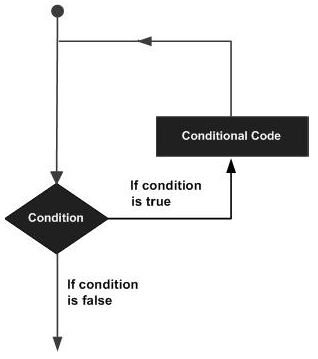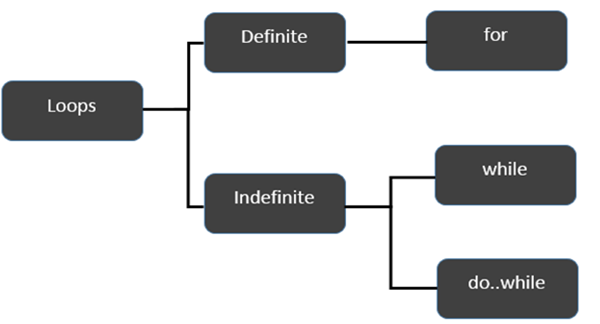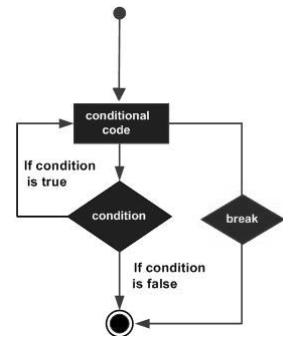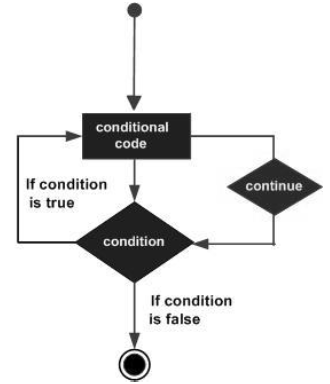# TypeScript 循环

2019-01-03 17:01 更新TypeScript提供了不同类型的循环来处理循环要求。下图说明了循环的分类：1for循环

for循环是一个确定循环的实现。

### 不确定循环

1while循环

2do...while循环

do ... while循环类似于while循环，只是do ... while循环不会在第一次循环执行时评估条件。

### 示例：while与do..while

```var n:number = 5
while(n > 5) {
console.log("Entered while")
}
do {
console.log("Entered do…while")
}
while(n>5)
```

```//Generated by typescript 1.8.10
var n = 5;
while (n > 5) {
console.log("Entered while");
}

do {
console.log("Entered do…while");
} while (n > 5);
```

```Entered do…while
```

## break语句

break语句用于将控件从结构中取出。在循环中使用break会导致程序退出循环。它的语法如下：

```break
```

### 流程图### 示例

```var i:number = 1
while(i<=10) {
if (i % 5 == 0) {
console.log ("The first multiple of 5  between 1 and 10 is : "+i)
break     //exit the loop if the first multiple is found
}
i++
}  //outputs 5 and exits the loop
```

```//Generated by typescript 1.8.10
var i = 1;

while (i <= 10) {
if (i % 5 == 0) {
console.log("The first multiple of 5  between 1 and 10 is : " + i);
break; //exit the loop if the first multiple is found
}
i++;
} //outputs 5 and exits the loop
```

```The first multiple of 5  between 1 and 10 is : 5
```

## continue语句

continue语句跳过当前迭代中的后续语句，并将控件带回循环的开头。与break语句不同，continue不会退出循环。它终止当前迭代并开始后续迭代。

### 语法

```continue
```

### 流程图### 示例

```var num:number = 0
var count:number = 0;

for(num=0;num<=20;num++) {
if (num % 2==0) {
continue
}
count++
}
console.log (" The count of odd values between 0 and 20 is: "+count)    //outputs 10
```

```//Generated by typescript 1.8.10
var num = 0;
var count = 0;

for (num = 0; num <= 20; num++) {
if (num % 2 == 0) {
continue;
}
count++;
}
console.log(" The count of odd values between 0 and 20 is: " + count);     //outputs 10
```

### 输出

```The count of odd values between 0 and 20 is: 10
```

## 无限循环

### 语法：使用for循环的无限循环

```for(;;) {
//statements
}示例：使用for循环的无限循环```
```for(;;) {
console.log(“This is an endless loop”)
}
```

### 语法：使用while循环的无限循环

```while(true) {
//statements
}
```

### 示例：使用while循环的无限循环

```while(true) {
console.log(“This is an endless loop”)
}```

App下载